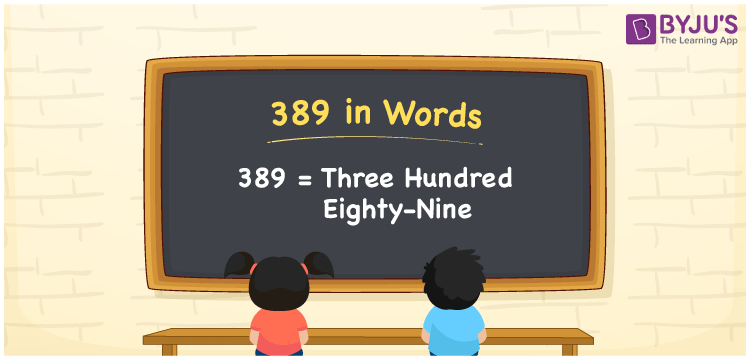# 389 in Words

389 in words is written as Three hundred eighty-nine. In both the International System of Numerals and the Indian System of Numerals, 389 is written as Three hundred eighty-nine. The number 389 is a Cardinal Number as it represents some quantity. For example, “the box weighs 389 grams”.

 389 in Words Three hundred eighty-nine Three hundred eighty-nine in Number 389

## 389 in English Words

We write 389 in English Words using the letters of the English alphabet. Therefore, we read 389 in English as “Three hundred eighty-nine.”## How to Write 389 in Words?

To write 389 in words, we shall use the place value chart. In the place value chart, write 3 in the hundreds, 8 in the tens, and 9 in the ones, respectively. Now let us make a place value chart to write the number 389 in words.

 Hundreds Tens Ones 3 8 9

Thus, we can write the expanded form as

3 × Hundred + 8 × Ten + 9 × One

= 3 × 100 + 8 × 10 + 9 × 1

= 300 + 80 + 9

= 389

= Three hundred eighty-nine.

389 is a natural number, the successor of 388 and the predecessor of 390.

389 in words – Three hundred eighty-nine

• Is 389 an odd number? – Yes
• Is 389 an even number? – No
• Is 389 a perfect square number? – No
• Is 389 a perfect cube number? – No
• Is 389 a prime number? – Yes
• Is 389 a composite number? – No

## Frequently Asked Questions on 389 in Words

Q1

### How to write 389 in words?

389 in words is written as Three hundred eighty-nine.
Q2

### How to write 389 in the International and Indian System of Numerals?

In both, the system of numerals, 389 in words, is written as Three hundred eighty-nine.
Q3

### What is the preceding number of 389?

The number that precedes 389 is 388.